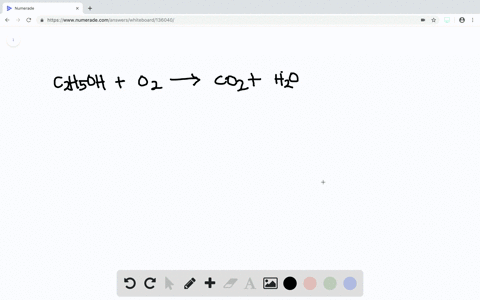Sign up for our free STEM online summer camps starting June 1st!View Summer Courses### The complete combustion of ethanol, $\mathrm{C}_{… 02:42Manhattan College Need more help? Fill out this quick form to get professional live tutoring. Get live tutoring Problem 38 A gas is confined to a cylinder under constant atmospheric pressure, as illustrated in Figure$5.4 .$When 0.49 kJ of heat is added to the gas, it expands and does 214 J of work on the surroundings. What are the values of$\Delta H$and$\Delta E\$ for this process?

$$\Delta H=0.49 \mathrm{KJ}$$

## Discussion

You must be signed in to discuss.

## Video Transcript

Okay, guys, we're doing problem number thirty eight of chapter five and cameras here. Central science. So the question is, a gas is confined to a soldier under constant atmospheric pressure. You could see using a figure five point four when point for nine killed, use of heat is at the gas expands and does two hundred fourteen jewels of work on surroundings where the values of Delta that the end of the age for this process. So we have our point four nine and kill Jules Air Heat, and we have our negative to fourteen Jules of work. Since we're doing work on this rises and not the system, we have to have a negative negative work is very straightforward Problem. Remember, Delta E is equal to que plus w you so so the e is equal to to your point for nine minus zero point two one four, t e is equal to zero point two seven six. Jules. Now, Delta age is equal to so as so we're dealing at a system at come on as as a constant Stackhouse and pressure. So we have constant constant p and at constant p p. D. Q. For as the Delta H is going to be equal to the our heat is going to be equal to Q. That means that Delta age that's going to be equal to equal to shit us. Just mistake here. This should be in killer Jules, not in jewels. Toe. So that easy eight point two seven six Killa Jules, not jewels. My apologies. And that the ages point for nine. Kill it, Jules.